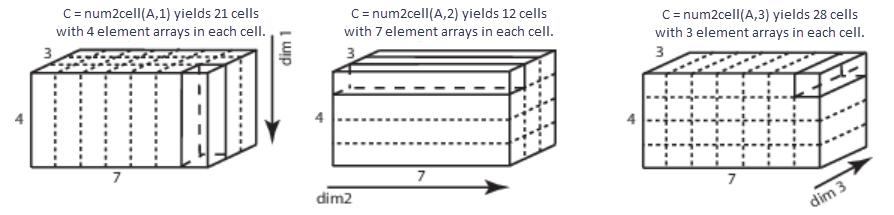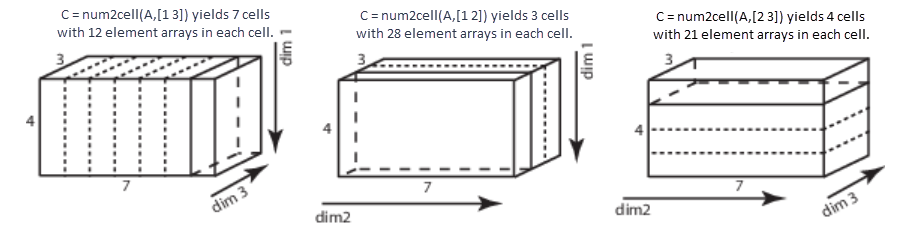# num2cell

## 语法

``C = num2cell(A)``
``C = num2cell(A,dim)``

## 说明

``C = num2cell(A)` 通过将 `A` 的每个元素放置于 `C` 的一个单独元胞中，来将数组 `A` 转换为元胞数组 `C`。`num2cell` 函数转换具有任意数据类型（甚至是非数值类型）的数组。`

````C = num2cell(A,dim)` 将 `A` 的内容划分成 `C` 中单独的元胞，其中 `dim` 指定每个元胞包含 `A` 的哪个维度。`dim` 可以是维度的标量或向量。例如，如果 `A` 有 2 行 3 列，那么： `num2cell(A,1)` 创建一个 1×3 元胞数组 `C`，其中每个元胞包含 `A` 的 2×1 列。`num2cell(A,2)` 创建一个 2×1 元胞数组 `C`，其中每个元胞包含 `A` 的 1×3 行。`num2cell(A,[1 2])` 创建一个 1×1 元胞数组 `C`，其中每个元胞包含 `A` 整个数组。 ```

## 示例

`a = magic(3)`
```a = 3×3 8 1 6 3 5 7 4 9 2 ```
`c = num2cell(a)`
```c=3×3 cell array {} {} {} {} {} {} {} {} {} ```

`a = ['four';'five';'nine']`
```a = 3x4 char array 'four' 'five' 'nine' ```
`c = num2cell(a)`
```c = 3x4 cell {'f'} {'o'} {'u'} {'r'} {'f'} {'i'} {'v'} {'e'} {'n'} {'i'} {'n'} {'e'} ```

```A = reshape(1:12,4,3); A(:,:,2) = A*10```
```A = A(:,:,1) = 1 5 9 2 6 10 3 7 11 4 8 12 A(:,:,2) = 10 50 90 20 60 100 30 70 110 40 80 120 ```
`C = num2cell(A,1)`
```C = 1x3x2 cell array C(:,:,1) = {4x1 double} {4x1 double} {4x1 double} C(:,:,2) = {4x1 double} {4x1 double} {4x1 double} ```

`C{1}`
```ans = 4×1 1 2 3 4 ```

`C = num2cell(A,2)`
```C = 4x1x2 cell array C(:,:,1) = {[ 1 5 9]} {[2 6 10]} {[3 7 11]} {[4 8 12]} C(:,:,2) = {[ 10 50 90]} {[20 60 100]} {[30 70 110]} {[40 80 120]} ```

`C{1}`
```ans = 1×3 1 5 9 ```

`C = num2cell(A,3)`
```C=4×3 cell array {1x1x2 double} {1x1x2 double} {1x1x2 double} {1x1x2 double} {1x1x2 double} {1x1x2 double} {1x1x2 double} {1x1x2 double} {1x1x2 double} {1x1x2 double} {1x1x2 double} {1x1x2 double} ```

`C{1}`
```ans = ans(:,:,1) = 1 ans(:,:,2) = 10 ```

```A = reshape(1:12,4,3); A(:,:,2) = A*10```
```A = A(:,:,1) = 1 5 9 2 6 10 3 7 11 4 8 12 A(:,:,2) = 10 50 90 20 60 100 30 70 110 40 80 120 ```
`c = num2cell(A,[1 3])`
```c=1×3 cell array {4x1x2 double} {4x1x2 double} {4x1x2 double} ```

`c{1}`
```ans = ans(:,:,1) = 1 2 3 4 ans(:,:,2) = 10 20 30 40 ```
`c = num2cell(A,[2 3])`
```c=4×1 cell array {1x3x2 double} {1x3x2 double} {1x3x2 double} {1x3x2 double} ```

## 输入参数

`A` 的维度，指定为正整数或正整数向量。`dim` 必须介于 1 和 `ndims`(`A`) 之间。

## 输出参数

• 如果未指定 `dim`，则 `C` 的大小与 `A` 相同。

• 如果 `dim` 是标量，则 `C` 包含 `numel(A)/size(A,dim)` 元胞。如果 `dim` 是 1 或 2，则每个元胞分别包含一个列向量或行向量。如果 `dim` > 2，则每个元胞包含一个第 `dim` 维的长度为 `size(A,dim)` 的数组，并且其他维度都是单一维度。

例如，给定一个 4×7×3 数组 `A`，此图窗将显示 `num2cell` 如何创建与 `dim``1``2``3` 相对应的元胞。• 如果 `dim` 是包含 `N` 个值的向量，则 `C` 具有 `numel(A)/prod([size(A,dim(1)),...,size(A,vdim(N))])` 个元胞。每个元胞都包含一个第 `dim``(i)` 维的长度为 `size(A,dim(i))` 并且其他维度为单一维度的数组。

例如，给定一个 4×7×3 数组，您可以将 `dim` 指定为一个正整数向量以创建不同维度的元胞数组。